# Join endpoints of multiple polylines to common point## Recommended PostsDear all,

I am looking for a lisp routine that join endpoints of multiple polylines to common point. I do not need to join them into one polyline, just touch all in one point.

Based on selection I want to get "master point" of first polyline in selection and then connect all the rest of polylines to that point as shown in attached picture.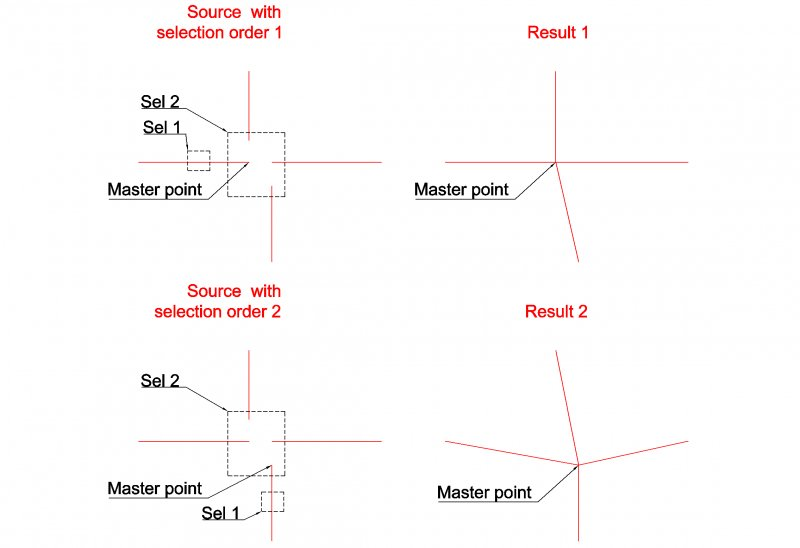##### Share on other sitesHere you go...

```(defun c:foo (/ cp p s x)
;; RJP » 2018-08-15
;; Puts plines and lines closest vertice to a common picked point
(cond
((and (setq p (getpoint "\nPick common point: "))
(setq s (ssget "_:L" '((0 . "LINE,LWPOLYLINE"))))
)
(foreach e	(vl-remove-if 'listp (mapcar 'cadr (ssnamex s)))
(setq cp	(car (vl-sort (list (vlax-curve-getstartpoint e) (vlax-curve-getendpoint e))
'(lambda (r j) (< (distance r p) (distance j p)))
)
)
)
(entmod (mapcar '(lambda	(x)
(cond	((equal (list (car cp) (cadr cp)) (cdr x) 1e- (cons (car x) p))
(x)
)
)
(entget e '("*"))
)
)
)
)
)
(princ)
)```

##### Share on other sitesHere you go...

```(defun c:foo (/ cp p s x)
;; RJP » 2018-08-15
;; Puts plines and lines closest vertice to a common picked point
(cond
((and (setq p (getpoint "\nPick common point: "))
(setq s (ssget "_:L" '((0 . "LINE,LWPOLYLINE"))))
)
(foreach e	(vl-remove-if 'listp (mapcar 'cadr (ssnamex s)))
(setq cp	(car (vl-sort (list (vlax-curve-getstartpoint e) (vlax-curve-getendpoint e))
'(lambda (r j) (< (distance r p) (distance j p)))
)
)
)
(entmod (mapcar '(lambda	(x)
(cond	((equal (list (car cp) (cadr cp)) (cdr x) 1e- (cons (car x) p))
(x)
)
)
(entget e '("*"))
)
)
)
)
)
(princ)
)```

Perfect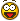Thank you.

I was struggling with polylines extended data but without success.

Could you modify it to be possible to apply it also at POLYLINES ?

Do not loose the time if it is more tricky.

##### Share on other sitesHere's another - this doesn't account for UCS which are not parallel to WCS:

```([color=BLUE]defun[/color] c:cpt ( [color=BLUE]/[/color] a b e i p q s x )
([color=BLUE]if[/color]
([color=BLUE]and[/color]
([color=BLUE]setq[/color] s
([color=BLUE]ssget[/color] [color=MAROON]"_:L"[/color]
'(
(-04 . [color=MAROON]"<OR"[/color])
(000 . [color=MAROON]"LINE"[/color])
(-04 . [color=MAROON]"<AND"[/color])
(000 . [color=MAROON]"*POLYLINE"[/color])
(-04 . [color=MAROON]"<NOT"[/color])
(-04 . [color=MAROON]"&="[/color])
(070 . 1)
(-04 . [color=MAROON]"NOT>"[/color])
(-04 . [color=MAROON]"AND>"[/color])
(-04 . [color=MAROON]"OR>"[/color])
)
)
)
([color=BLUE]setq[/color] p ([color=BLUE]getpoint[/color] [color=MAROON]"\nSpecify common point: "[/color]))
([color=BLUE]setq[/color] p ([color=BLUE]trans[/color] p 1 0))
)
([color=BLUE]repeat[/color] ([color=BLUE]setq[/color] i ([color=BLUE]sslength[/color] s))
([color=BLUE]setq[/color] i ([color=BLUE]1-[/color] i)
e ([color=BLUE]ssname[/color] s i)
x ([color=BLUE]entget[/color] e)
)
([color=BLUE]if[/color] ([color=BLUE]=[/color] [color=MAROON]"POLYLINE"[/color] ([color=BLUE]cdr[/color] ([color=BLUE]assoc[/color] 0 x)))
([color=BLUE]progn[/color]
([color=BLUE]setq[/color] e ([color=BLUE]entnext[/color] e)
x ([color=BLUE]entget[/color]  e)
a x
)
([color=BLUE]while[/color] ([color=BLUE]=[/color] [color=MAROON]"VERTEX"[/color] ([color=BLUE]cdr[/color] ([color=BLUE]assoc[/color] 0 x)))
([color=BLUE]setq[/color] b x
e ([color=BLUE]entnext[/color] e)
x ([color=BLUE]entget[/color]  e)
)
)
([color=BLUE]setq[/color] q ([color=BLUE]if[/color] ([color=BLUE]<[/color] ([color=BLUE]distance[/color] p ([color=BLUE]cdr[/color] ([color=BLUE]assoc[/color] 10 a))) ([color=BLUE]distance[/color] p ([color=BLUE]cdr[/color] ([color=BLUE]assoc[/color] 10 b)))) a b))
([color=BLUE]if[/color] ([color=BLUE]entmod[/color] ([color=BLUE]subst[/color] ([color=BLUE]cons[/color] 10 p) ([color=BLUE]assoc[/color] 10 q) q))
([color=BLUE]entupd[/color] ([color=BLUE]cdr[/color] ([color=BLUE]assoc[/color] 330 q)))
)
)
([color=BLUE]apply[/color]
'([color=BLUE]lambda[/color] ( a b [color=BLUE]/[/color] q )
([color=BLUE]setq[/color] q ([color=BLUE]if[/color] ([color=BLUE]<[/color] ([color=BLUE]distance[/color] p ([color=BLUE]cdr[/color] a)) ([color=BLUE]distance[/color] p ([color=BLUE]cdr[/color] b))) a b))
([color=BLUE]if[/color] ([color=BLUE]entmod[/color] ([color=BLUE]subst[/color] ([color=BLUE]cons[/color] ([color=BLUE]car[/color] q) p) q x))
([color=BLUE]entupd[/color] e)
)
)
([color=BLUE]if[/color] ([color=BLUE]=[/color] [color=MAROON]"LINE"[/color] ([color=BLUE]cdr[/color] ([color=BLUE]assoc[/color] 0 x)))
([color=BLUE]list[/color] ([color=BLUE]assoc[/color] 10 x) ([color=BLUE]assoc[/color] 11 x))
([color=BLUE]list[/color] ([color=BLUE]assoc[/color] 10 x) ([color=BLUE]assoc[/color] 10 ([color=BLUE]reverse[/color] x)))
)
)
)
)
)
([color=BLUE]princ[/color])
)
```

##### Share on other sitesCouldn't you also use constraints to maintain that endpoint to endpoint relationship?

##### Share on other sitesNice Lee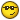##### Share on other sitesNice LeeThanks Ron - you too - I like the concision of your code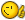##### Share on other sitesThanks Ron - you too - I like the concision of your codeCheers!##### Share on other sitesHere's another - this doesn't account for UCS which are not parallel to WCS:

```([color=BLUE]defun[/color] c:cpt ( [color=BLUE]/[/color] a b e i p q s x )
([color=BLUE]if[/color]
([color=BLUE]and[/color]
([color=BLUE]setq[/color] s
([color=BLUE]ssget[/color] [color=MAROON]"_:L"[/color]
'(
(-04 . [color=MAROON]"<OR"[/color])
(000 . [color=MAROON]"LINE"[/color])
(-04 . [color=MAROON]"<AND"[/color])
(000 . [color=MAROON]"*POLYLINE"[/color])
(-04 . [color=MAROON]"<NOT"[/color])
(-04 . [color=MAROON]"&="[/color])
(070 . 1)
(-04 . [color=MAROON]"NOT>"[/color])
(-04 . [color=MAROON]"AND>"[/color])
(-04 . [color=MAROON]"OR>"[/color])
)
)
)
([color=BLUE]setq[/color] p ([color=BLUE]getpoint[/color] [color=MAROON]"\nSpecify common point: "[/color]))
([color=BLUE]setq[/color] p ([color=BLUE]trans[/color] p 1 0))
)
([color=BLUE]repeat[/color] ([color=BLUE]setq[/color] i ([color=BLUE]sslength[/color] s))
([color=BLUE]setq[/color] i ([color=BLUE]1-[/color] i)
e ([color=BLUE]ssname[/color] s i)
x ([color=BLUE]entget[/color] e)
)
([color=BLUE]if[/color] ([color=BLUE]=[/color] [color=MAROON]"POLYLINE"[/color] ([color=BLUE]cdr[/color] ([color=BLUE]assoc[/color] 0 x)))
([color=BLUE]progn[/color]
([color=BLUE]setq[/color] e ([color=BLUE]entnext[/color] e)
x ([color=BLUE]entget[/color]  e)
a x
)
([color=BLUE]while[/color] ([color=BLUE]=[/color] [color=MAROON]"VERTEX"[/color] ([color=BLUE]cdr[/color] ([color=BLUE]assoc[/color] 0 x)))
([color=BLUE]setq[/color] b x
e ([color=BLUE]entnext[/color] e)
x ([color=BLUE]entget[/color]  e)
)
)
([color=BLUE]setq[/color] q ([color=BLUE]if[/color] ([color=BLUE]<[/color] ([color=BLUE]distance[/color] p ([color=BLUE]cdr[/color] ([color=BLUE]assoc[/color] 10 a))) ([color=BLUE]distance[/color] p ([color=BLUE]cdr[/color] ([color=BLUE]assoc[/color] 10 b)))) a b))
([color=BLUE]if[/color] ([color=BLUE]entmod[/color] ([color=BLUE]subst[/color] ([color=BLUE]cons[/color] 10 p) ([color=BLUE]assoc[/color] 10 q) q))
([color=BLUE]entupd[/color] ([color=BLUE]cdr[/color] ([color=BLUE]assoc[/color] 330 q)))
)
)
([color=BLUE]apply[/color]
'([color=BLUE]lambda[/color] ( a b [color=BLUE]/[/color] q )
([color=BLUE]setq[/color] q ([color=BLUE]if[/color] ([color=BLUE]<[/color] ([color=BLUE]distance[/color] p ([color=BLUE]cdr[/color] a)) ([color=BLUE]distance[/color] p ([color=BLUE]cdr[/color] b))) a b))
([color=BLUE]if[/color] ([color=BLUE]entmod[/color] ([color=BLUE]subst[/color] ([color=BLUE]cons[/color] ([color=BLUE]car[/color] q) p) q x))
([color=BLUE]entupd[/color] e)
)
)
([color=BLUE]if[/color] ([color=BLUE]=[/color] [color=MAROON]"LINE"[/color] ([color=BLUE]cdr[/color] ([color=BLUE]assoc[/color] 0 x)))
([color=BLUE]list[/color] ([color=BLUE]assoc[/color] 10 x) ([color=BLUE]assoc[/color] 11 x))
([color=BLUE]list[/color] ([color=BLUE]assoc[/color] 10 x) ([color=BLUE]assoc[/color] 10 ([color=BLUE]reverse[/color] x)))
)
)
)
)
)
([color=BLUE]princ[/color])
)
```

Thnks Lee.

It works perfectly##### Share on other sitesYou're welcome Jozef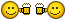## Join the conversation

You can post now and register later. If you have an account, sign in now to post with your account.
Note: Your post will require moderator approval before it will be visible.×   Pasted as rich text.   Restore formatting

Only 75 emoji are allowed.